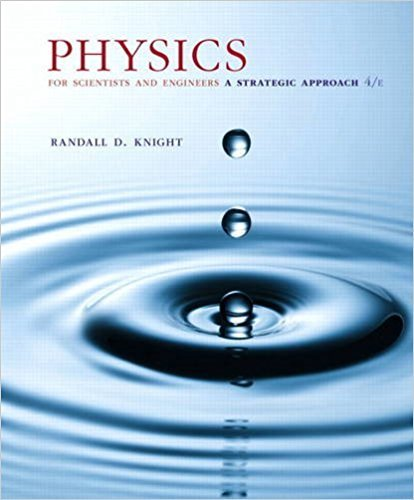# The resistivity of a metal increases slightly with## Problem 27.82 Chapter 27

Physics for Scientists and Engineers: A Strategic Approach, Standard Edition (Chs 1-36) | 4th Edition

• 2901 Step-by-step solutions solved by professors and subject experts
• Get 24/7 help from StudySoup virtual teaching assistantsPhysics for Scientists and Engineers: A Strategic Approach, Standard Edition (Chs 1-36) | 4th Edition

4 5 0 363 Reviews
20
0
Problem 27.82

The resistivity of a metal increases slightly with increased temperature. This can be expressed as r = r0 31 + a1T - T024, where T0 is a reference temperature, usually 20C, and a is the temperature coefficient of resistivity. a. First find an expression for the current I through a wire of length L, cross-section area A, and temperature T when connected across the terminals of an ideal battery with terminal voltage V. Then, because the change in resistance is small, use the binomial approximation to simplify your expression. Your final expression should have the temperature coefficient a in the numerator. b. For copper, a = 3.9 * 10-3 C-1 . Suppose a 2.5-m-long, 0.40-mm-diameter copper wire is connected across the terminals of a 1.5 V ideal battery. What is the current in the wire at 20C? c. What is the rate, in A/C, at which the current changes with temperature as the wire heats up?

Step-by-Step Solution:
Step 1 of 3

Lecture 7 Potential from a distribution of charges X V = 1 qi 4⇡✏0 ri §Smooth distribution 1 X qi 1 Z ⇢ V = = dV 4⇡✏0 i ri 4⇡✏ 0 r § of point charges is usually much simpler thanup calculating the electric field •It’s a scalar Electric Potential from Two Oppositely Charged Point Charges §The electric field lines from two...

Step 2 of 3

Step 3 of 3

##### ISBN: 9780134081496

Since the solution to 27.82 from 27 chapter was answered, more than 228 students have viewed the full step-by-step answer. Physics for Scientists and Engineers: A Strategic Approach, Standard Edition (Chs 1-36) was written by and is associated to the ISBN: 9780134081496. This full solution covers the following key subjects: . This expansive textbook survival guide covers 42 chapters, and 4463 solutions. The answer to “The resistivity of a metal increases slightly with increased temperature. This can be expressed as r = r0 31 + a1T - T024, where T0 is a reference temperature, usually 20C, and a is the temperature coefficient of resistivity. a. First find an expression for the current I through a wire of length L, cross-section area A, and temperature T when connected across the terminals of an ideal battery with terminal voltage V. Then, because the change in resistance is small, use the binomial approximation to simplify your expression. Your final expression should have the temperature coefficient a in the numerator. b. For copper, a = 3.9 * 10-3 C-1 . Suppose a 2.5-m-long, 0.40-mm-diameter copper wire is connected across the terminals of a 1.5 V ideal battery. What is the current in the wire at 20C? c. What is the rate, in A/C, at which the current changes with temperature as the wire heats up?” is broken down into a number of easy to follow steps, and 156 words. This textbook survival guide was created for the textbook: Physics for Scientists and Engineers: A Strategic Approach, Standard Edition (Chs 1-36), edition: 4. The full step-by-step solution to problem: 27.82 from chapter: 27 was answered by , our top Physics solution expert on 12/28/17, 08:06PM.

Unlock Textbook Solution

The resistivity of a metal increases slightly with

×
Get Full Access to Physics For Scientists And Engineers: A Strategic Approach, Standard Edition (Chs 1 36) - 4 Edition - Chapter 27 - Problem 27.82

Get Full Access to Physics For Scientists And Engineers: A Strategic Approach, Standard Edition (Chs 1 36) - 4 Edition - Chapter 27 - Problem 27.82

I don't want to reset my password

Need help? Contact support

Need an Account? Is not associated with an account
We're here to help